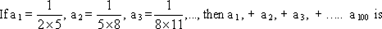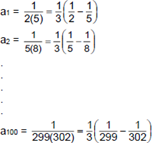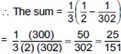# CAT 2017 – Slot 2 – Quantitative Ability – If f(ab) = f(a)f(b) for all positive integers a and b

Q. 1: If f(ab) = f(a)f(b) for all positive integers a and b, then the largest possible value of f(l) is

Let us take the case when a=b=1

So, f(1) = f(1) f(1)

f(1) = [f(1)]^2

f(1)[f(1)-1] = 0

f(1) = 1

So, the maximum value of f(1) = 1

##### Q. 2: Let f(x) = 2x-5and g(x) = 7-2x. Then |f(x) + g(x)| = |f(x)| + |g(x)| if and only ifA) 5/2 < x < 7/2B) x ≤ 5/2 or x ≥ 7/2C) x < 5/2 or x ≥ 7/2D) 5/2 ≤ x ≤ 7/2

|f(x) + g(x)| = |f(x)| + |g(x)|

Putting value of f(x) and g(x), we get,

|2x-5| + |7-2x| = 2

1st Case: When x<=5/2

-2x + 5 +7 – 2x = 2

=> x=5/2

2nd Case: 5/2 < x < 7/2

On solving, we get, 2=2, which satisfies the condition

3rd Case: x ≥ 7/2

2x-5 – 7+2x = 2

x=7/2

So, the answer should be 5/2<= x <= 7/2

Option (D)

Q. 3: An infinite geometric progression a1, a2, a3,… has the property that an = 3(a(n+ l) + a(n+2) +….) for every n ≥ 1. If the sum a1 + a2 + a3 +……. = 32, then a5 is
A) 1/32
B) 2/32
C) 3/32
D) 4/32

For any n ≥ 1, an = 3 (a(n+1) + a(n+2) + ……..)

So, a1 = 3 (a2 + a3 + ……) or r = 1/4 and

a1 + a2 + a3 +………… = 4a1/3 = 32

So, a1 = 24

GP = 24, 6, 1.5,…….

a5 = 1.5/16 = 3/32

Option (C)

Q. 4:A) 25/151
B) 1/2
C) 1/4
D) 111/55

Explanation:-All the terms like 1/5, 1/8,… 1/299 will cancel out##### Checkout Other Questions of CAT 2017 Slot 2 Paper:

Verbal Ability :              |   Q.01- Q.06  |  Q.07- Q.12  |  Q.13- Q.18  |  Q.19- Q.21  |  Q.22- Q.24  |  Q.25- Q.29  |  Q.30 – Q.34  |

Logical Reasoning :    |   Q.29 – Q.32  |

Quantitative Aptitude: |

### Free Material Area